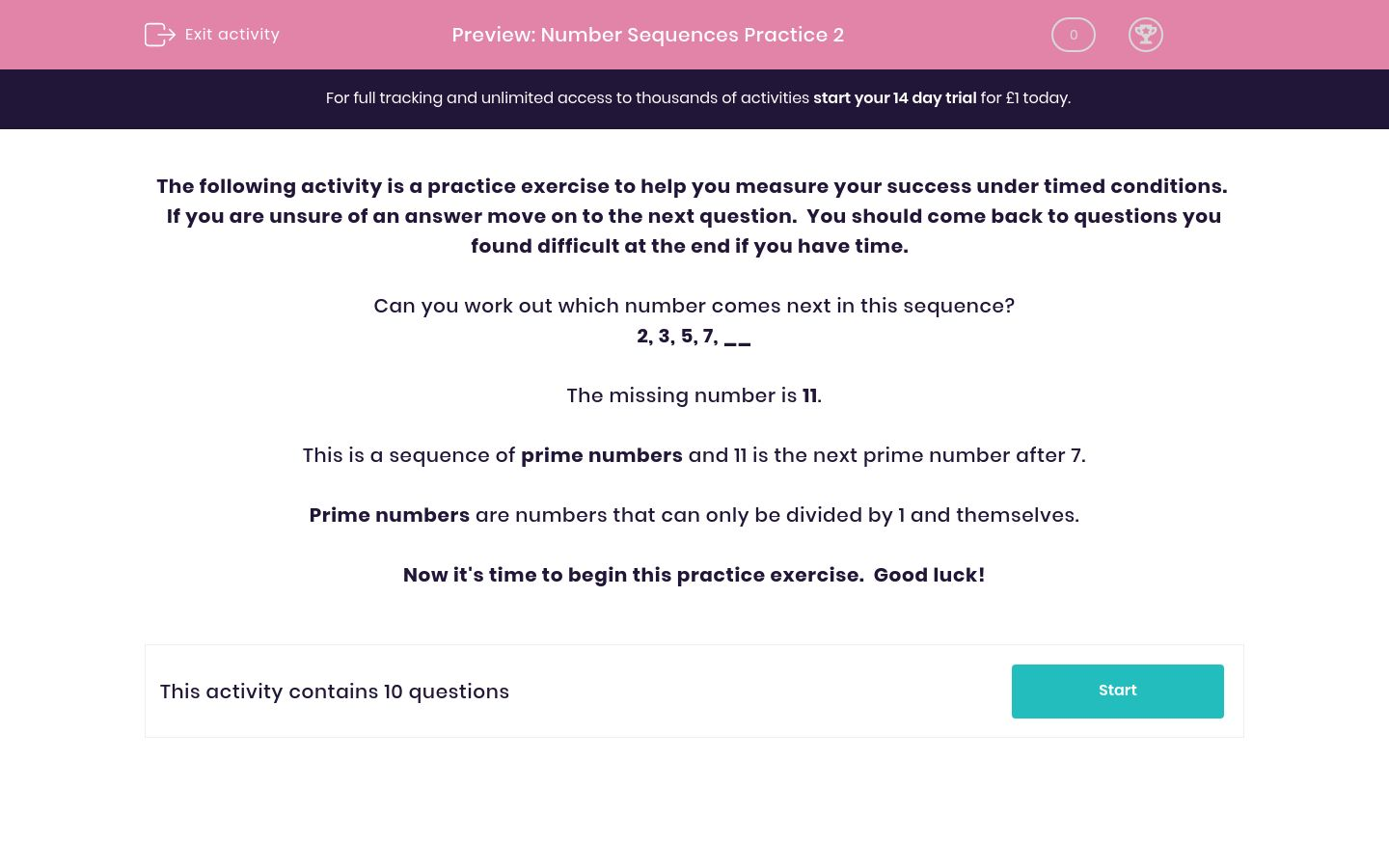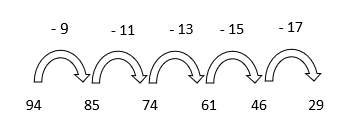# Number Sequences Practice 2

In this worksheet, students will identify missing numbers in a range of complex sequences, including prime, square and triangle numbers.Key stage:  KS 2

Curriculum topic:   Exam-Style Questions: Verbal Reasoning

Curriculum subtopic:   Exam-Style Questions: Number Sequences

Difficulty level:### QUESTION 1 of 10

If you are unsure of an answer move on to the next question.  You should come back to questions you found difficult at the end if you have time.

Can you work out which number comes next in this sequence?

2, 3, 5, 7, __

The missing number is 11.

This is a sequence of prime numbers and 11 is the next prime number after 7.

Prime numbers are numbers that can only be divided by 1 and themselves.

Now it's time to begin this practice exercise.  Good luck!

Choose the number that best completes the sequence.

5, 15, 45, 135, __

305

270

405

135

150

Which number would come next in the following sequence?

94, 85, 74, 61, 46, __

29

41

31

25

33

Which number comes next in the following sequence?

1, 3, 6, 10, 15, __

20

21

19

17

18

Which number continues the pattern in this number sequence?

178, 160, 144, 130, 118, __110

112

108

120

100

Which number would continue the pattern below?

225, 196, 169, 144, 121, __

81

144

121

100

72

Which number would continue the pattern below?

96, 79, 62, 45, 28, __

13

11

17

21

12

Which number would continue the pattern below?

15, 21, 27, 33, __

37

38

39

40

41

Which number would come next in the following sequence?

58, 2, 52, 4, 46, 6, __

45

72

50

40

3

Which number would come next in the following sequence?

100, 99, 95, 86, 70, __

45

72

49

50

63

Which number would come next in the following sequence?

2, 6, 4, 8, 6, __

12

10

11

6

8

• Question 1

Choose the number that best completes the sequence.

5, 15, 45, 135, __

405
EDDIE SAYS
Hey number detective! In our sequence here, the numbers are multiplying by 3 each time. To work out the next number in the sequence, we need to multiply 135 by 3. 135 x 3 = 405. Top tip: Use a piece of paper to label your jumps and find a relationship between the numbers.
• Question 2

Which number would come next in the following sequence?

94, 85, 74, 61, 46, __

29
EDDIE SAYS
We had a changing number sequence here. This means that the amount we were taking away each time was changing. Did you label those jumps? If we label the jumps we can see that we are taking away 9, then 11, then 13, then 15. What do you notice about these numbers? They are increasing in odd numbers. This means that we need to take away 17 next. 46 - 17 = 29. This makes 29 the next number in the sequence.• Question 3

Which number comes next in the following sequence?

1, 3, 6, 10, 15, __

21
EDDIE SAYS
Did you recognise our triangle number sequence here? The number that we add changes each time here.The last number we added was 5. This means we need to +6 to 15. This gives us the answer of 21. What would be the next triangle number in the sequence?
• Question 4

Which number continues the pattern in this number sequence?

178, 160, 144, 130, 118, __108
EDDIE SAYS
It's another changing number sequence! The numbers are decreasing by different even numbers each time. First we -18, then -16, then -14 and then -12. Can you spot the pattern and work out what we need to take away this time? We need to -10 as we are subtracting a smaller even number each time.• Question 5

Which number would continue the pattern below?

225, 196, 169, 144, 121, __

100
EDDIE SAYS
We had some challenging numbers here detective. Do you recognise the numbers 144 and 121? These are both square numbers. If we do some quick multiplication we can see that: 13 x 13 = 169 14 x 14 = 196 15 x 15 = 225 So we have a decreasing square number sequence here. The square number before 121 is 100. Well done if you got that tricky one!
• Question 6

Which number would continue the pattern below?

96, 79, 62, 45, 28, __

11
EDDIE SAYS
Did you carefully count between the numbers here? We are subtracting 17 each time. 17 less than 28 is 11. I'm counting on your careful counting here!
• Question 7

Which number would continue the pattern below?

15, 21, 27, 33, __

39
EDDIE SAYS
Did you spot the pattern here? We are adding 6 each time in this sequence. 33 + 6 = 39. Keep up the excellent sequence spotting detective!
• Question 8

Which number would come next in the following sequence?

58, 2, 52, 4, 46, 6, __

40
EDDIE SAYS
There were two hidden sequences in the number sequence above. Did you spot either of them? From the first number (58) we are taking away 6 but jumping over a number each time. From the second number (2) we are adding 2 but jumping over a number each time. To work out the next number in the sequence, we need to take away 6 from 46. This gives us 40.• Question 9

Which number would come next in the following sequence?

100, 99, 95, 86, 70, __

45
EDDIE SAYS
Here's another changing number sequence for you. First we -1, then -4, then -9 and then -16. What do you notice about the numbers that we are taking away each time? These are all square numbers. We need to take away 25 as this is the next square number. 70 - 25 = 45.• Question 10

Which number would come next in the following sequence?

2, 6, 4, 8, 6, __

10
EDDIE SAYS
Did you spot the rule for our sequence here? In this sequence, we are alternating between adding 4 and then subtracting 2. The rule is: +4 -2 +4 -2, etc. We need to add 4 to 6. This gives us the answer of 10. Excellent sequence spotting detective! Well done.
---- OR ----

Sign up for a £1 trial so you can track and measure your child's progress on this activity.

### What is EdPlace?

We're your National Curriculum aligned online education content provider helping each child succeed in English, maths and science from year 1 to GCSE. With an EdPlace account you’ll be able to track and measure progress, helping each child achieve their best. We build confidence and attainment by personalising each child’s learning at a level that suits them.

Get started